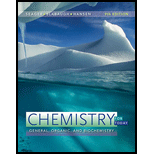Chapter 9, Problem 9.27EChemistry for Today: General, Orga...

9th Edition
Spencer L. Seager + 2 others
ISBN: 9781305960060

Solutions

Chapter
SectionChemistry for Today: General, Orga...

9th Edition
Spencer L. Seager + 2 others
ISBN: 9781305960060
Textbook Problem

Calculate the molar concentration of OH − in water solutions with the following H 3 O + molar concentrations:a. 1.0 × 10 − 6 b. 4.8 × 10 − 4 c. 6.2 × 10 − 10 d. 1.4 e. 0.038

Interpretation Introduction

(a)

Interpretation:

The molar concentration of OH in water solutions with the given H3O+ molar concentrations is to be calculated.

Concept introduction:

The water undergoes self ionization which can be represented by the reaction,

H2O(l)+H2O(l)H3O+(aq)+OH(aq)

The ionization constant of water is represented as,

K=[H3O+][OH][H2O][H2O]

The concentration of water remains constant and the self-ionization constant of water becomes,

Kw=[H3O+][OH]Kw=(1.0×107mol/L)(1.0×107mol/L)Kw=1.0×1014(mol/L)2

Explanation

The ionic product of water Kw is,

Kw=[H3O+][OH]

The value of Kw is 1.0×1014(mol/L)2.

The given H3O+ molar concentration is 1.0×106mol/L. Substitute this value in the formula for ionic product.

1.0×1014(mol/L)2=1

Interpretation Introduction

(b)

Interpretation:

The molar concentration of OH in water solutions with the given H3O+ molar concentrations is to be calculated.

Concept introduction:

The water undergoes self ionization which can be represented by the reaction,

H2O(l)+H2O(l)H3O+(aq)+OH(aq)

The ionization constant of water is represented as,

K=[H3O+][OH][H2O][H2O]

The concentration of water remains constant and the self-ionization constant of water becomes,

Kw=[H3O+][OH]Kw=(1.0×107mol/L)(1.0×107mol/L)Kw=1.0×1014(mol/L)2

Interpretation Introduction

(c)

Interpretation:

The molar concentration of OH in water solutions with the given H3O+ molar concentrations is to be calculated.

Concept introduction:

The water undergoes self ionization which can be represented by the reaction,

H2O(l)+H2O(l)H3O+(aq)+OH(aq)

The ionization constant of water is represented as,

K=[H3O+][OH][H2O][H2O]

The concentration of water remains constant and the self-ionization constant of water becomes,

Kw=[H3O+][OH]Kw=(1.0×107mol/L)(1.0×107mol/L)Kw=1.0×1014(mol/L)2

Interpretation Introduction

(d)

Interpretation:

The molar concentration of OH in water solutions with the given H3O+ molar concentrations is to be calculated.

Concept introduction:

The water undergoes self ionization which can be represented by the reaction,

H2O(l)+H2O(l)H3O+(aq)+OH(aq)

The ionization constant of water is represented as,

K=[H3O+][OH][H2O][H2O]

The concentration of water remains constant and the self-ionization constant of water becomes,

Kw=[H3O+][OH]Kw=(1.0×107mol/L)(1.0×107mol/L)Kw=1.0×1014(mol/L)2

Interpretation Introduction

(e)

Interpretation:

The molar concentration of OH in water solutions with the given H3O+ molar concentrations is to be calculated.

Concept introduction:

The water undergoes self ionization which can be represented by the reaction,

H2O(l)+H2O(l)H3O+(aq)+OH(aq)

The ionization constant of water is represented as,

K=[H3O+][OH][H2O][H2O]

The concentration of water remains constant and the self-ionization constant of water becomes,

Kw=[H3O+][OH]Kw=(1.0×107mol/L)(1.0×107mol/L)Kw=1.0×1014(mol/L)2

Still sussing out bartleby?

Check out a sample textbook solution.

See a sample solution

The Solution to Your Study Problems

Bartleby provides explanations to thousands of textbook problems written by our experts, many with advanced degrees!

Get Started

Find more solutions based on key concepts